# 5+ Formulas to Find Duplicates in One Column in Excel

Finding duplicates is a very common task in an Excel spreadsheet. This article presents more than 5 formulas to find duplicates in one column in Excel.

## 1st Formula to Find Duplicates in One Column in Excel

What this formula actually does?

The formula returns TRUE if the content is a duplicate. Otherwise, it returns FALSE.

The COUNTIF function is a combined version of the COUNT function and the IF function. We can use the COUNTIF function to easily find the duplicates in one column in Excel.

Syntax

`COUNTIF(range, criteria)`

Usage Guide

Step_1: Insert the formula in cell C2.

`=COUNTIF(\$B\$2:\$B\$15,B2)>1`

Step_2: Press ENTER.

This will return the formula result in cell C2.

Step_3: Select cells C2 to C15.

Step_4: With all the cells being selected, press CTRL + D.

This will copy the above formula in all the selected cells with changing cell references.

Final Result >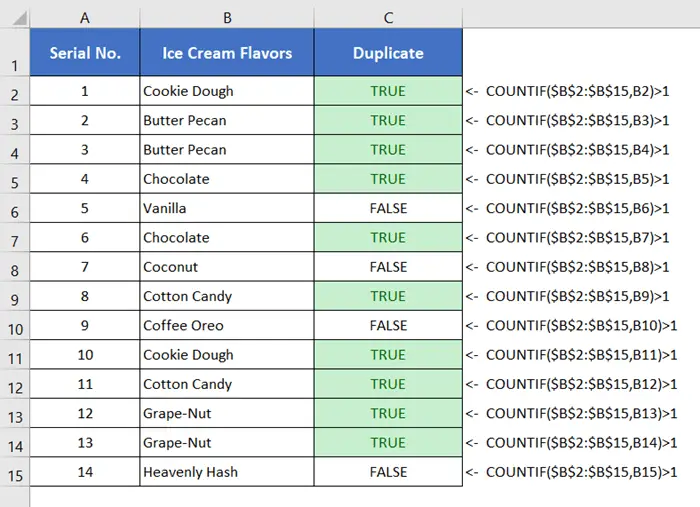Formula Explanation

• \$B\$2:\$B\$15 is the cell range where we want to find the duplicates.
• B2 is the criteria here which is the first cell in the range B2:B15.

The COUNTIF function compares the content of cell B2 with that of the range B2:B15. If any content appears more than once, it treats that content as a duplicate value.

As the range is fixed here, we must lock the range. We can use absolute cell references by imposing dollar signs (\$) before the cell references to lock the range.

🔗 How to Find Duplicates in Two Columns in Excel (7 Methods)

## 2nd Formula to Find Duplicates in One Column in Excel

What this formula actually does?

The formula returns Duplicate when it finds duplicate content. Otherwise, it returns Unique.

The previous formula returns TRUE for the duplicate values and FALSE for the unique values.

But if you want to display other messages in exchange for TRUE or FALSE, you need to merge one extra function with the previous formula.

Syntax

`IF(COUNTIF(range, criteria),value_if_true,value_if_false)`

Usage Guide

Step_1: Type the formula in cell C2.

`=IF(COUNTIF(\$B\$2:\$B\$15,B2)>1,"Duplicate","Unique")`

Step_2: Press ENTER to insert the formula.

Step_3: Select the range C2 to C15.

Step_4: Select the Home tab > Editing group > Fill drop-down menu > Down.

This will copy down the above formula to the selected range with changing cell references.

Final Result >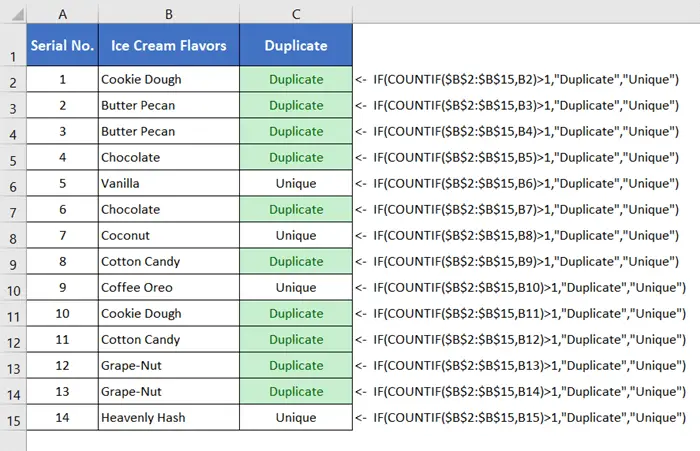Formula Explanation

• \$B\$2:\$B\$15 is the cell range where we want to find the duplicates. As the range is fixed here, we must lock the range.
• B2 is the criteria here which is the first cell in the range B2:B15.

The COUNTIF function compares the content of cell B2 with that of the range B2:B15. If any content appears more than once, the COUNTIF function returns TRUE. Otherwise, it returns FALSE.

When the COUNTIF function returns TRUE, the IF function returns Duplicate. But when the COUNTIF function returns FALSE, the IF function returns Unique. This the how the formula works.

🔗 How to Compare Rows in Excel for Duplicates (7 Ways)

🔗 How to Find Similar Text in Two Columns in Excel (5 Ways)

## 3rd Formula to Find Duplicates in One Column in Excel

What this formula actually does?

This formula skips the first appearance of a duplicate value. It returns Duplicate when it finds duplicate contents. Otherwise, it returns Unique.

The above two formulas identify the first appearance of a duplicate value as a Duplicate.

But if you want to skip the first appearance of the duplicate value and only identity the second and onward appearances, then you can use this formula.

Syntax

`IF(COUNTIF(range, criteria),value_if_true,value_if_false)`

Usage Guide

Everything is the same as the previous_method. Just use the following formula instead of the formula used in the previous method.

`=IF(COUNTIF(\$B\$2:\$B2,B2)>1,"Duplicate","Unique")`

Final Result >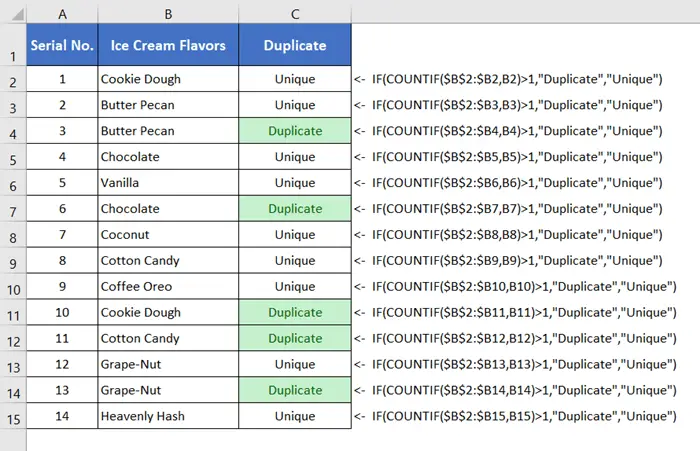Formula Explanation

Just look at the range (\$B\$2:\$B2) inside the COUNTIF function. The first cell of this range is locked up. Only the second cell of the range can be changed to \$B2, \$B3, \$B4, etc.

For the first appearance of a value, COUNTIF(\$B\$2:\$B2,B2)>1 becomes false. Thus, it returns FALSE. As a result, the IF function returns Unique.

For the second and onward appearance of a value, COUNTIF(\$B\$2:\$B2,B2)>1 becomes TRUE. Thus, it returns TRUE. As a result, the IF function returns Duplicate.

🔗 5 Ways to Find Matching Values in Two Worksheets in Excel

🔗 Find, Highlight, and Remove Duplicates in Excel [Step-by-Step]

## 4th Formula to Find Duplicates in Excel

What this formula actually does?

This formula returns the sequence of a duplicate value’s appearances in terms of 1,2,3, etc.

Syntax

`COUNTIF(range, criteria)`

Formula

`=COUNTIF(\$B\$2:\$B\$15,B2)`

To read the usage guide and explanation of this formula, click_here.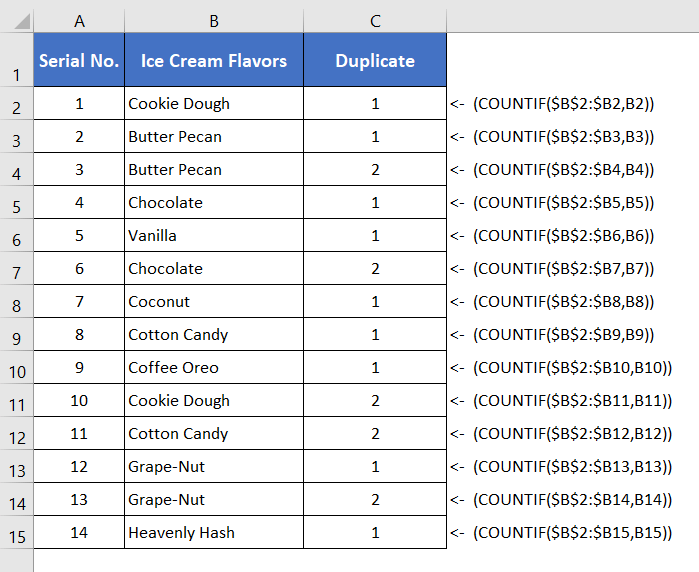🔗 4+ Methods to Filter Duplicate Values in Excel

## 5th Formula to Find Duplicates in Excel

What this formula actually does?

This is a case-sensitive formula. It returns “Duplicate” when a certain value has more than one exact appearance. Otherwise, it returns “Unique”.

Syntax

`IF(SUM(--EXACT(range, criteria))),value_if_true,value_if_false)`

Formula

`=IF(SUM((--EXACT(\$B\$2:\$B\$15,B2)))<=1,"Unique","Duplicate")`

Read the usage guide of the formula in Excel.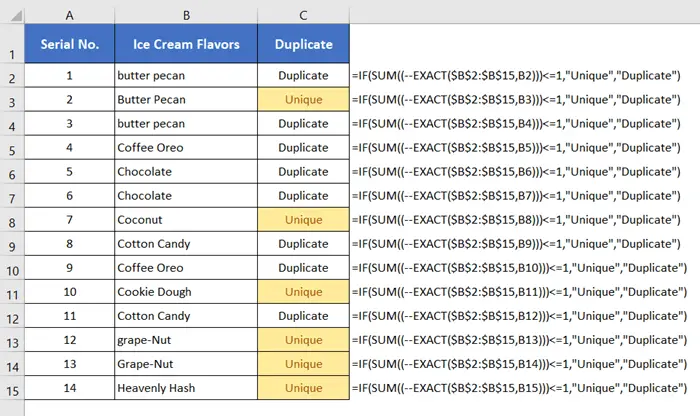Formula Explanation

The EXACT function looks for exact matches between the content of cell B2 with that of the range \$B\$2:\$B\$15. The SUM function calculates the summation of the number of times the EXACT function finds a match. If the summation becomes less than or equal to 1, the IF function returns “Unique”. Otherwise, it returns “Duplicate”.

🔗 How to Remove Duplicates in Excel [13 + Different Methods]

## Find Duplicates in One Column Based on Condition (Another Column)

What this formula actually does?

This formula finds the duplicates in one column based on criteria set in another column.

In this particular example, the formula discussed below can find the duplicates in the column, Ice Cream Flavors. In the column, Company, two ice cream manufacturer company name is enlisted. The formula will find duplicates of ice cream flavor names under the same company.

Syntax

`IF(COUNTIFS(criteria_range1, criteria1, [criteria_range2, criteria2]…),value_if_true,value_if_false)`

Formula

`=IF(COUNTIFS(\$A\$2:\$A\$15,A2,\$B\$2:\$B\$15,B2)>1,"","Unique")`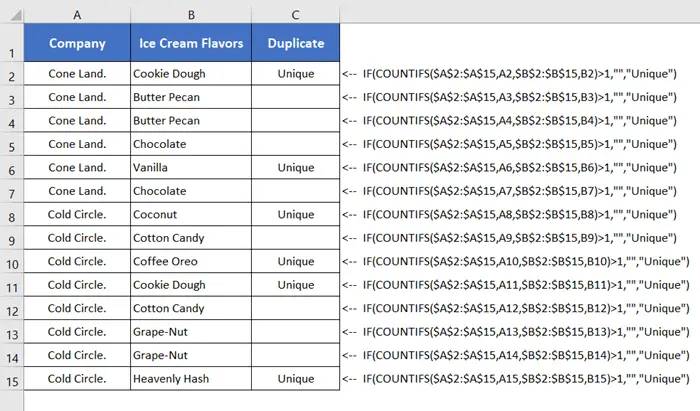Formula Explanation

• \$A\$2:\$A\$15: This is The cell range of the column, Company.
• A2: This is the first cell of criteria_range1.
• \$B\$2:\$B\$15: This is The cell range of the column, Ice Cream Flavors.
• B2: This is the first cell of criteria_range2.

🔗 4+ Ways to Find Duplicates in a Column and Delete Rows in Excel

🔗 Vlookup for Duplicate Values and Return the Matches in Excel [8 Cases]

## Conclusion

I’ve discussed more than 5 formulas to find the duplicates in one column in Excel. I expect you’ve found this article useful. You can read more articles relating to Excel from the Blog page of our website.